# Frequency Domain Analysis and Associated Demosaicking Algorithms for the Diagonal Stripe Structure

This page describes in detail the luma-chroma demultiplexing formulation for demosaicking of signals sampled with the diagonal stripe CFA of section 7.3.3.2, along with the results of the least-squares filter design, organized as shown below. Software is provided to allow these results to be reproduced.

### Contents:

Geometric structure of the Diagonal stripe color-filter array

Formation and representation of the Diagonal stripe CFA image

Demosaicking algorithms derived from the frequency-domain representation using luma-chroma demultiplexing

Least-squares filter design for CFA signal demultiplexing

Least-squares luma-chroma demultiplexing (LSLCD) algorithm results for the stripe pattern

LSLCD optimization - quality vs complexity

## Geometric structure of the Diagonal stripe color-filter array

The geometric structure of the diagonal stripe pattern is similar to that presented in the sample below: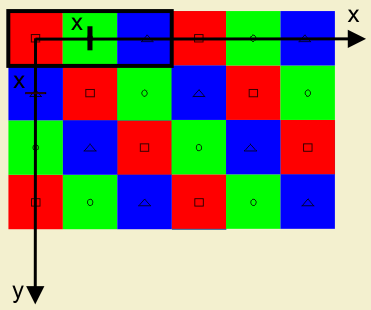The Diagonal stripe pattern is regular and periodic. It lies on an integer lattice Λ = ℤ2. The horizontal and vertical sampling spaces in this samping structure are equal. The distance between them is X, used as the unit of length, pixel height (px).

The Diagonal stripe structure has three classes corresponding to the red (R), green (G) and blue (B) filters. The origin of the set is at the upper left corner with a red sample. The red, green and blue pattern is repeated periodically. The nearest same-color sample is found on the lower right corner of each sample. One period of the Stripe pattern determined by the sublattice Γ is indicated by the bold contour in the upper left corner.

## Formation and representation of the Diagonal stripe CFA image

Following the chapter's theory, one can describe the Diagonal stripe pattern using matrices.

• The sampling matrix Λ and the sublattice Γ representing a period of the pattern can be written as: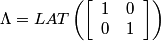,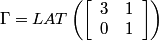.

• The coset representatives of Γ in Λ can be defined by: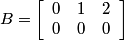.

• The color channels are represented by: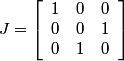.

In the frequency domain the Diagonal stipe pattern has the following characteristics:

• The reciprocal lattices Λ* in Γ* are: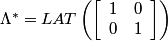,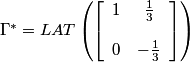.

• The reciprocal lattices Λ* (⊙) and Γ* (+) and a suitable choice of representatives for the cosets of Λ* in Γ* are represented below.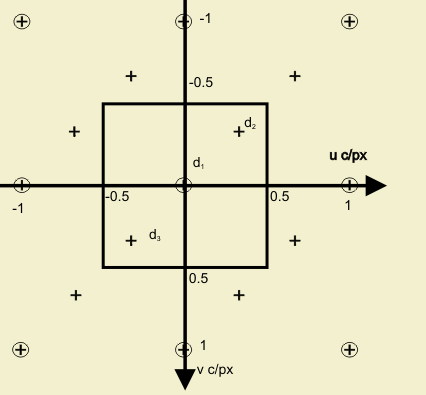• A suitable choice of coset representatives of Λ* in Γ* gives: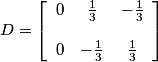.

Considering the choices made above, we obtain the following :

• The coefficient matrix M is: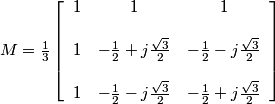.

• This means that the three transformed signals are: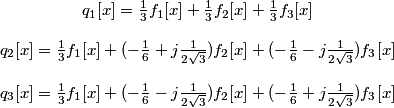.

Here, we see that q2[x]and q3[x] are complex and q3[x] = q2*[x].

The inverse transformation recovers the original components using the matrix M-1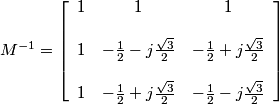.

In the frequency domain FCFA, becomes: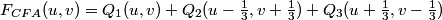.

The use of complex signals can be avoided by expressing the modulation as a quadrature modulation of real signals. Rather than considering the two modulated signals: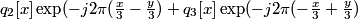we can consider the equivalent real, quadrature modulated signals: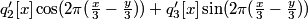where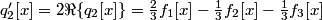and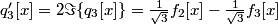.

One period of the two-dimensional power density spectrum of a sample CFA image with the diagonal stripe CFA structure is shown below.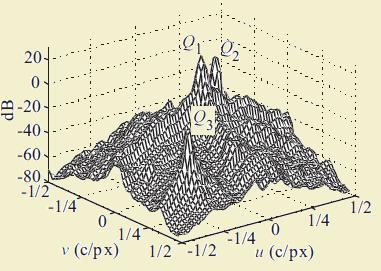The three different components are identified in the figure.

## Demosaicking algorithms derived from the frequency-domain representation using luma-chroma demultiplexing

The idea behind demosaicking algorithms based on the frequency-domain representation is to extract the luma and modulated chrominance components from the CFA signal using spatial filters and then to transform the demodulated chrominance components to the desired tristimulus values.

In the case of the Diagonal stripe pattern, the demosaicking algorithm is straightforward:

1. Filter fCFA with a complex bandpass filter h2 centered at frequency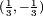to extract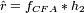, and shift it to baseband to estimate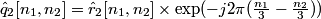.

2. Estimate the luma component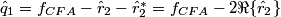3. Estimate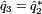4. Estimate the RGB components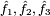from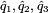In practice, the input and output signals are real, and the complex operations above can be expanded to obtain the real version of the algorithm: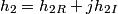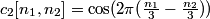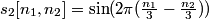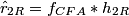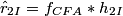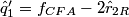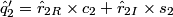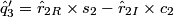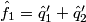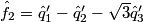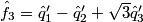The block diagram of the demultiplexing algorithm for the stripe structure is represented below: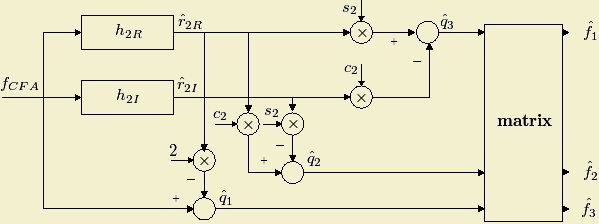## Least-squares filter design for CFA signal demultiplexing

The LSLCD algorithm for the diagonal stripe structure follows exactly the approach in the chapter.

An example of designed filters can be seen here.

For our testing, we used different training sets:

• the image itself
• the other 23 images of the Kodak set (for each image)
• all the 24 images of the Kodak set

## LSLCD algorithm results for the stripe pattern

A full list of results for the 24 Kodak images can be found here.

The table below shows the LSLCD algorithm results for the first 3 Kodak images. The training set chosen here is all the 24 Kodak images. :

 No. Thumb Original LSLCD 1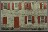TIFF TIFF JPG 36.87 2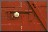TIFF TIFF JPG 38.93 3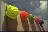TIFF TIFF JPG 39.46

## LSLCD optimization - quality vs complexity

The quality of the demultiplexed image and the complexity of the filters used in the algorithm are directly related. The quality versus complexity plot for the diagonal stripe pattern is below. The optimization algorithm details and the related observations can be found here.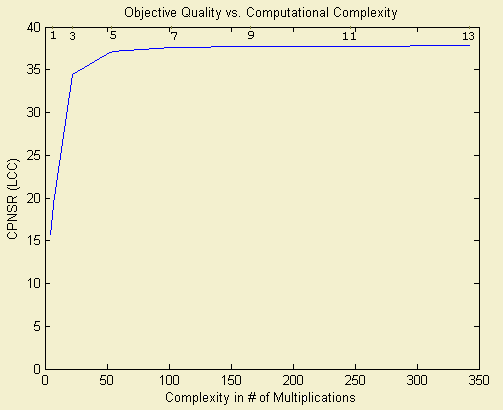The recommended filter size is 5x5.